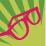# Failing to create polygon geometry via the Python API

780
6
07-24-2017 10:59 PMNew Contributor III

Hi guys, I'm trying to add a feature to AGOL via the Python API. My features are being created but with empty geometries. At the bottom is my geometry object that I am passing through. The geometry was created using the following so should be fine:

point = arcpy.Point(float(x),float(y))
sr = arcpy.SpatialReference(2193)
ptGeometry = arcpy.PointGeometry(point,sr)
buff_geometry = ptGeometry.buffer(length)
densify = buff_geometry.densify('DISTANCE',10,10)
shape = densify.JSON

I'm sure someone has pushed polygon objects through to AGOL via the Python API but I cannot find any code samples to compare mine too. Any help appreciated - also does the API handle true curves? Or do I need to use densify? Thanks! Greg.

{'geometry': '{"rings":[[[1795330.473,5541901.9229999995],[1795604.8544855313,5541877.9177305344],[1795870.8990284686,5541806.6313101035],[1796120.5229999998,5541690.2297405191],[1796346.1417020655,5541532.2498245714],[1796540.899824572,5541337.4917020649],[1796698.8797405194,5541111.8729999997],[1796815.2813101034,5540862.2490284685],[1796886.5677305341,5540596.204485531],[1796910.5729999996,5540321.8229999999],[1796886.5677305341,5540047.4415144688],[1796815.2813101034,5539781.3969715312],[1796698.8797405194,5539531.773],[1796540.899824572,5539306.1542979348],[1796346.1417020655,5539111.3961754283],[1796120.5229999998,5538953.4162594806],[1795870.8990284686,5538837.0146898963],[1795604.8544855313,5538765.7282694653],[1795330.473,5538741.7230000002],[1795056.0915144687,5538765.7282694653],[1794790.0469715314,5538837.0146898963],[1794540.4230000002,5538953.4162594806],[1794314.8042979345,5539111.3961754283],[1794120.046175428,5539306.1542979348],[1793962.0662594805,5539531.773],[1793845.6646898966,5539781.3969715312],[1793774.3782694659,5540047.4415144688],[1793750.3730000004,5540321.8229999999],[1793774.3782694659,5540596.204485531],[1793845.6646898966,5540862.2490284685],[1793962.0662594805,5541111.8729999997],[1794120.046175428,5541337.4917020649],[1794314.8042979345,5541532.2498245714],[1794540.4230000002,5541690.2297405191],[1794790.0469715314,5541806.6313101035],[1795056.0915144687,5541877.9177305344],[1795330.473,5541901.9229999995]]],"spatialReference":{"wkid":2193,"latestWkid":2193}}'

Tags (3)
1 Solution

Accepted SolutionsNew Contributor III

Solved it - needed the last line that loads the JSON format. Turns out that the JSON property returns a string not a JSON format.

``        point = arcpy.Point(float(x),float(y))        sr = arcpy.SpatialReference(2193)        ptGeometry = arcpy.PointGeometry(point,sr)        buff_geometry = ptGeometry.buffer(length)        densify = buff_geometry.densify('DISTANCE',1,1)        shape = densify.JSON        shape = json.loads(shape)‍‍‍‍‍‍‍``
6 RepliesbyMVP Esteemed Contributor

The code you posted is ArcPy, not ArcGIS API for Python.  Are you trying to use the ArcGIS API for Python to pass the geometry to AGOL or some other means?  If the former, what is that Python code you are using?

Neither ArcPy nor ArcGIS API for Python has its own geometry model, they both implement Esri's geometry object model.  Esri's geometry object model supports true curves, but that doesn't mean each API that implements the geometry object model supports true curves, or supports them well.

ArcPy partially supports true curves in that it implements Esri geometry object model, but many of the ArcPy functions and methods don't know how to work with true curves, which can cause confusion when ArcPy code automagically converts a true curve into a linear approximation.

In your ArcPy code above, the circle returned from buffer will be a true curve, it is you calling the densify function that converts it to a linear approximation.

``>>> point = arcpy.Point(10., 10.)>>> sr = arcpy.SpatialReference(2193)>>> ptGeometry = arcpy.PointGeometry(point, sr)>>> buff_geometry = ptGeometry.buffer(5)>>> buff_geometry.JSONu'{"curveRings":[[[10,15],{"a":[[10,15],[10,10],0,1]}]],"spatialReference":{"wkid":2193,"latestWkid":2193}}'>>> ‍‍‍‍‍‍‍``New Contributor III

Hi Joshua, Yes I'm using arcpy to create the geometry that I am then passing to AGOL via the Python API.

I'm passing the dictionary below through. The Python API is creating my features with the correct attributes, but they have no geometry. I have tried with and without true curves (I thought true curves may be the issue) but to no avail.

``pivot_dict = {"attributes":                {"farmName": farmName,                "centreX": centreX,                "centreY": centreY},                "geometry": shape}‍‍‍‍‍‍‍‍‍‍‍‍‍‍‍‍‍‍‍‍‍‍‍‍‍``byMVP Esteemed Contributor

Although having the dictionary structure is helpful, without seeing more of the code to pass the object up to AGOL, I can't offer any other suggestions on using the Python API.New Contributor III

The only code to pass data through to AGOL is this line:

``add_result = pivots_layer.edit_features(adds = [pivot_dict])‍``

Which does work, except for the geometry being empty in AGOL.byMVP Regular Contributor

umm...

It looks like ArcGIS API for Python does not support the true curves.

In order to draw it on the web map, it needs to be converted using the densify function.New Contributor III

Solved it - needed the last line that loads the JSON format. Turns out that the JSON property returns a string not a JSON format.

``        point = arcpy.Point(float(x),float(y))        sr = arcpy.SpatialReference(2193)        ptGeometry = arcpy.PointGeometry(point,sr)        buff_geometry = ptGeometry.buffer(length)        densify = buff_geometry.densify('DISTANCE',1,1)        shape = densify.JSON        shape = json.loads(shape)‍‍‍‍‍‍‍``